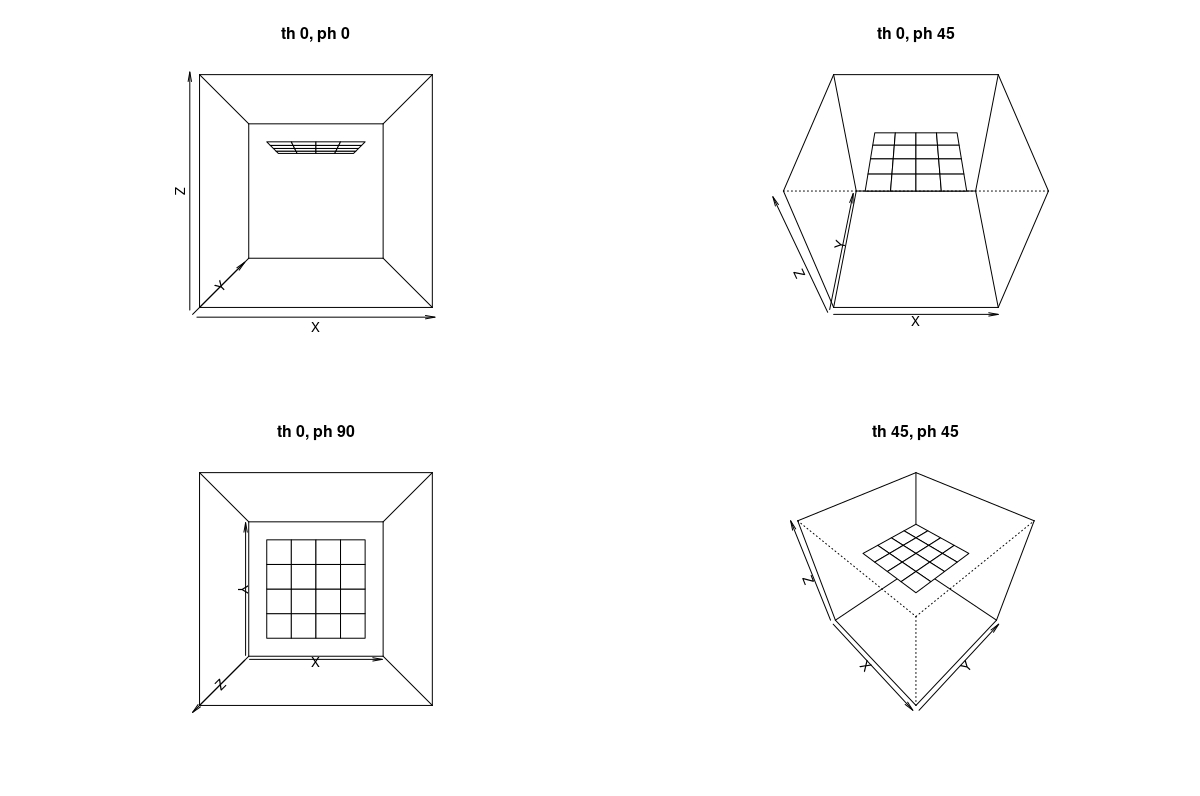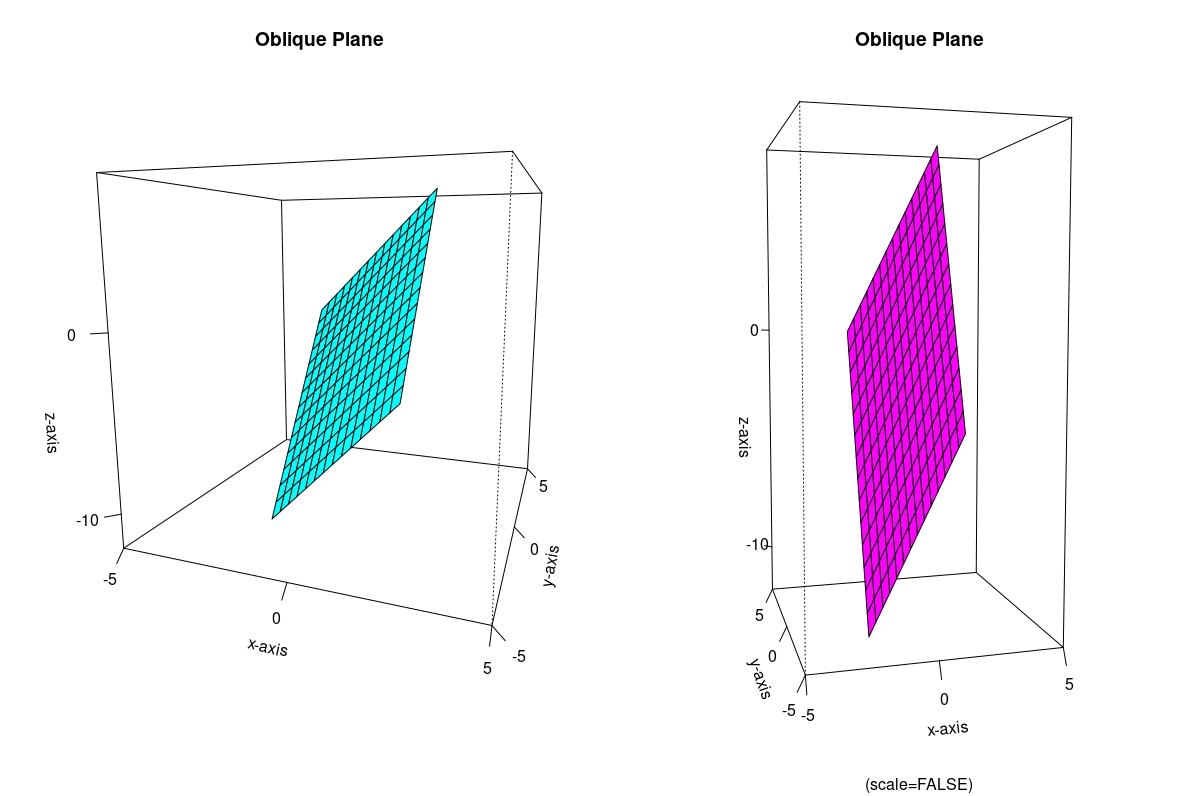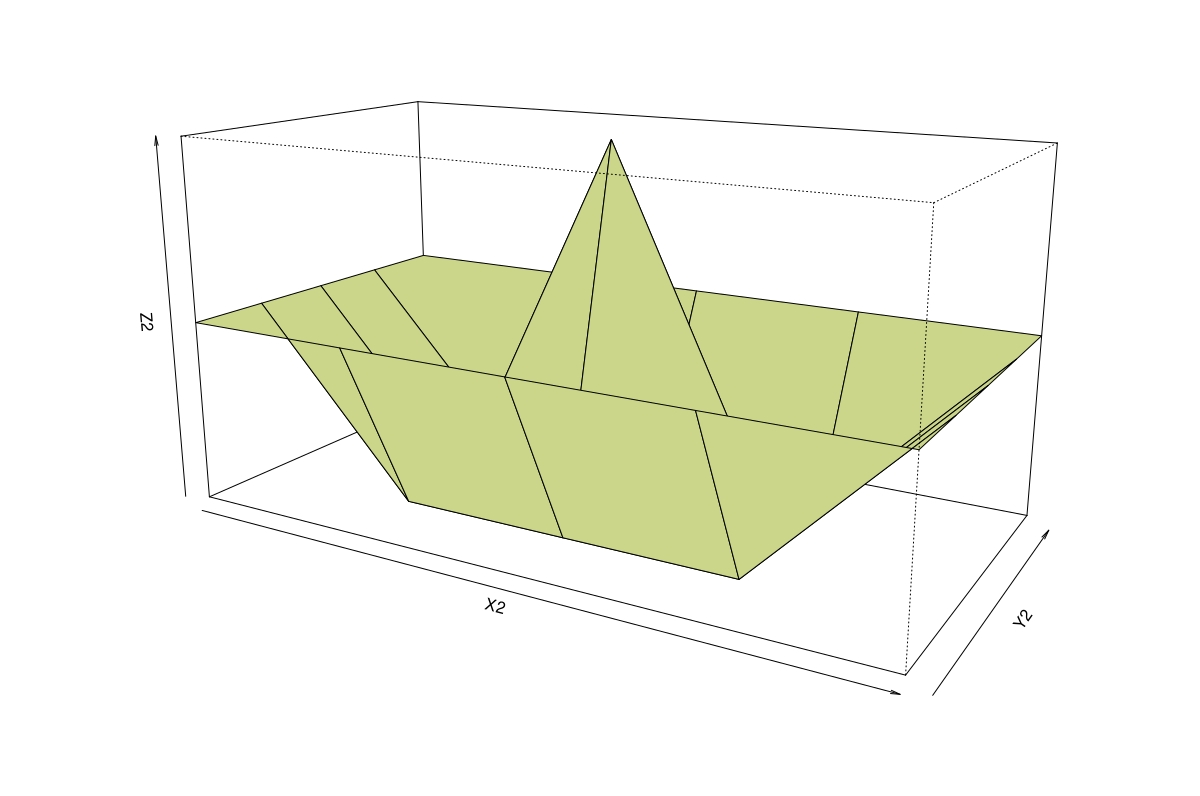# Create a 3D Perspective Plot in R

Jesse John May 24, 2022

We can create a perspective plot of a surface using base R’s `persp()` function. The surface passes through the `z` values corresponding to all pairs of `(x,y)` values.

To use the `persp()` function, we need to understand the format of its main arguments. The rest of this article demonstrates and explains these with simple examples.

## Arguments of the `persp()` Function in R

There are three arguments `x`, `y` and `z` for the 3 coordinates since `persp()` plots in 3 dimensions.

1. `x` and `y` are numeric vectors. These must be in ascending order.

2. The argument `z` takes a matrix. There must be one value of `z` in this matrix corresponding to each combination of `x` and `y`.

The surface that is plotted passes through all the `z` values.

One way to create the matrix for `z` is to use the `outer()` function to apply a bivariate function to all combinations of `x` and `y` values.

By default, the `persp()` function shows the view from the negative side of the `y`-axis.

1. The `phi` argument gives the colatitude. Changing `phi` from 0 to 90 degrees affects changing the view from the front to the top.
2. The `theta` argument gives the azimuth. Changing `theta` from 0 to 90 degrees has the effect of rotating the figure clockwise about its vertical axis.

## Plot of a Plane Surface in R

We will now create two planes and view them from different angles. The first is a horizontal plane.

Example Code:

``````# A function that gives a constant z value.
H = function(x, y){
return (0*x+0*y+1)
}

X = seq(-1,1,length.out=5)
Y = seq(-1,1, length.out=5)

# Apply the H function to all pairs (x,y).
# This gives the z matrix.
Z = outer(X, Y, H)

# Four different views.
persp(x=X,y=Y,z=Z, theta=0, phi=0, xlim=range(-2,2), ylim=range(-2,2), zlim=range(-2,2))
persp(x=X,y=Y,z=Z, theta=0, phi=45, xlim=range(-2,2), ylim=range(-2,2), zlim=range(-2,2))
persp(x=X,y=Y,z=Z, theta=0, phi=90, xlim=range(-2,2), ylim=range(-2,2), zlim=range(-2,2))
persp(x=X,y=Y,z=Z, theta=45, phi=45, xlim=range(-2,2), ylim=range(-2,2), zlim=range(-2,2))
``````

Output figures:The four figures illustrate the effect of changing the angles `theta` and `phi`.

Next, we will look at an oblique plane. The syntax also illustrates setting other `persp()` function arguments.

Example Code:

``````# A function for a plane.
O = function(x, y){
return (2*x+3*y-2)
}

X1 = seq(-2,2,length.out=15)
Y1 = seq(-2,2, length.out=15)

# Apply the function to all combinations of x and y.
Z1 = outer(X1, Y1, O)

# Check the range of the values of Z.
# This will help create the limits for the box.
range(Z1)

# Because we do not use the scale=FALSE argument, the output is a cube.
# The axes are scaled.
persp(x=X1,y=Y1,z=Z1, theta=20, phi=10, xlim=range(-5,5), ylim=range(-5,5), zlim=range(-12,8),
xlab="x-axis",
ylab="y-axis",
zlab="z-axis",
main="Oblique Plane",
col="#00FFFF",
ticktype="detailed",
nticks=3)

# With scale=FALSE.
persp(x=X1,y=Y1,z=Z1, theta=-15, phi=5, xlim=range(-5,5), ylim=range(-5,5), zlim=range(-12,8),
scale = FALSE,
xlab="x-axis",
ylab="y-axis",
zlab="z-axis",
main="Oblique Plane",
col="#FF00FF",
ticktype="detailed",
nticks=3)
``````

Output figures:## Plot from a Matrix in R

The `persp()` function can plot from a matrix of `z` values corresponding to vectors `x` and `y` in increasing order.

The number of rows and columns of `z` must match the lengths of `x` and `y`, respectively.

Example Code:

``````# Manually create a matrix of z values corresponding
# to all combinations of some x and y.
# (Or use such a pre-existing matrix.)
Z2 = matrix(data=c(1,1,1,1,1,
1,-1,-1,-1,1,
1,-1,3,-1,1,
1,-1,-1,-1,1,
1,1,1,1,1), ncol=5)

X2 = seq(-4, 4, length.out=5)
Y2 = seq(-2, 2, by=1)

persp(x=X2, y=Y2, z=Z2, scale=FALSE, col="#CBD68A", theta=30, phi=15)
``````

Output figure:Author: Jesse John

Jesse is passionate about data analysis and visualization. He uses the R statistical programming language for all aspects of his work.